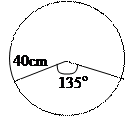Home Technical Mathematics Languages Science Social Science Art Literature Arabic Islamic Studies C.R.K HistoryMusicVisual Art Clothing/Textile Home Management Shorthand
 General Mathematics Paper 2, Nov/Dec. 2009
 Questions: 1 2 3 4 5 6 7 8 9 10 11 12 13 Main
Weakness/Remedies
Strength
Question 4

A sector of angle 135° is removed from a thin circular metal sheet of radius 40cm.
It is then folded with the straight edges coinciding to form a right circular cone.
Calculate the:

• base radius of the cone, correct to two decimal places
• greatest volume of liquid which the cone can hold, leaving your answer

correct to the nearest cm3.   (Take p = 22/7).

_____________________________________________________________________________________________________
Observation

The chief examiner reported that majority of the candidates attempted this question and many performed well.  However, some candidates could not interpret the relationship between a cone and an arc of a circle correctly.  While some candidates used the slant height of the cone as the height of the cone, others used the radius of the arc as the radius of the base of the cone.  A few other candidates were not able to draw the diagram correctly.This diagram would have aided them to establish that the length of the arc of the circle = circumference of the base of the cone and that the radius of the sector = slant height of the cone. i.e. 135/360 x 2 x p x 40/1 = 2 x p x r where r = base radius of cone. Simplifying gave r = 15cm.

Slant height of cone, = l  =  40cm, r = 15cm. Using pythagoras theorem,
h2 + r2 = l 2  where h = perpendicular height of cone.
\ h = √402 – 152 = 37.081 cm.  Therefore volume of Cone = 1/3 x p x r2 x h

1 x 22 x 15 x 15 x 37.081   =  8741cm3 to the nearest cm3.
3     7          1             1